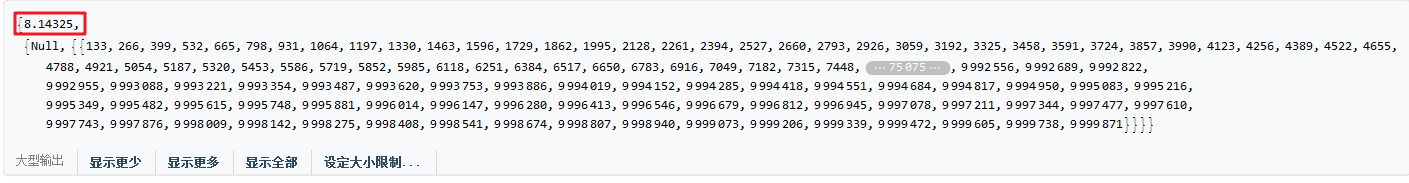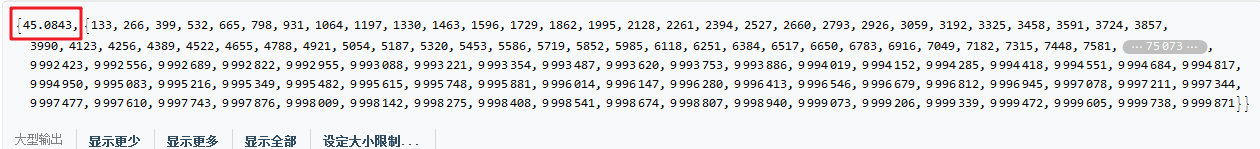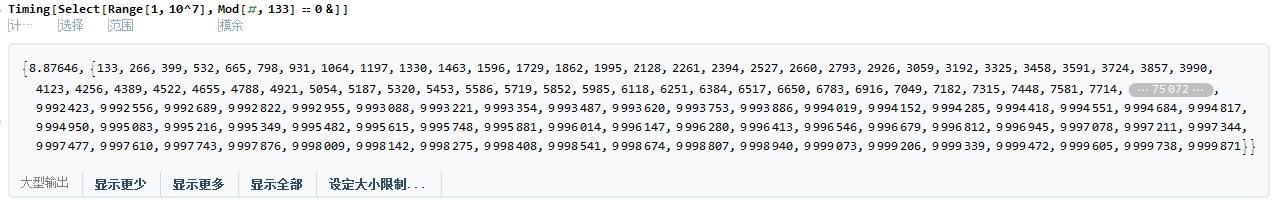# Mathematica优化AppendTo–Reap&Sow

• A+

## Reap&Sow基本用法

``````Reap[Sow[a = 3]; a += Sow[a^2 + 1]; Sow[a]; a = Sqrt[a + a^2]]
>> {Sqrt, {{3, 10, 13}}}``````

## Reap&Sow与AppendTo对比

### Reap&Sow

``````Timing[
Reap[
Do[(If[Mod[i, 133] == 0, Sow[i]]), {i, 1, 10^7}]
]
]``````### AppendTo

``````Timing[
t = {};
Do[
(If[Mod[i, 133] == 0, AppendTo[t, i]]), {i, 1, 10^7}
];
t
]````````Timing[Select[Range[1, 10^7], Mod[#, 133] == 0 &]]``• 微信公众号
• 关注微信公众号
•• QQ群
• 我们的QQ群号
•